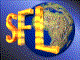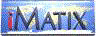| iMatix home page | << | < | > | >>SFLVersion 2.10

### strmatch

```#include "sflstr.h"
int
strmatch (
const char *string1,
const char *string2)
```

#### Synopsis

Calculates a similarity index for the two strings. This is a value from 0 to 32767 with higher values indicating a closer match. The two strings are compared without regard for case. The algorithm was designed by Leif Svalgaard leif@ibm.net.

#### Source Code - (sflstr.c)

```{
static int
name_weight  = {
20, 15, 13, 11, 10, 9, 8, 8, 7, 7, 7, 6, 6, 6, 6,
6,  5,  5,  5,  5, 5, 4, 4, 4, 4, 4, 4, 4, 4, 4
};
int
comp_index,
name_index,
start_of_string,
longest_so_far,
substring_contribution,
substring_length,
compare_length,
longest_length,
length_difference,
name_length,
char_index,
similarity_index,
similarity_weight;
char
cur_name_char;

ASSERT (string1);
ASSERT (string2);

name_length    = strlen (string1);
compare_length = strlen (string2);
if (name_length > compare_length)
{
length_difference = name_length - compare_length;
longest_length    = name_length;
}
else
{
length_difference = compare_length - name_length;
longest_length    = compare_length;
}
if (compare_length)
{
similarity_weight = 0;
substring_contribution = 0;

for (char_index = 0; char_index < name_length; char_index++)
{
start_of_string = char_index;
cur_name_char   = (char) tolower (string1 [char_index]);
longest_so_far  = 0;
comp_index      = 0;

while (comp_index < compare_length)
{
while ((comp_index < compare_length)
&&     (tolower (string2 [comp_index]) != cur_name_char))
comp_index++;

substring_length = 0;
name_index = start_of_string;

while ((comp_index < compare_length)
&&     (tolower (string2 [comp_index])
== tolower (string1 [name_index])))
{
if (comp_index == name_index)
substring_contribution++;
comp_index++;
if (name_index < name_length)
{
name_index++;
substring_length++;
}
}
substring_contribution += (substring_length + 1) * 3;
if (longest_so_far < substring_length)
longest_so_far = substring_length;
}
similarity_weight += (substring_contribution
+ longest_so_far + 1) * 2;
similarity_weight /= name_length + 1;
}
similarity_index  = (name_length < 30? name_weight [name_length]: 3)
* longest_length;
similarity_index /= 10;
similarity_index += 2 * length_difference / longest_length;
similarity_index  = 100 * similarity_weight / similarity_index;
}
else
similarity_index = 0;

return (similarity_index);
}
```

 | << | < | > | >>Copyright © 1996-99 iMatix Corporation